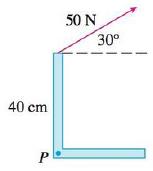Chapter 12, Problem 14RE

Chapter
Section
Textbook Problem

# Find the magnitude of the torque about P if a 50-N force is applied as shown.To determine

To find: The magnitude of the torque about P.

Explanation

Given:

|F|=50N and |r|=40cm.

Formula:

Write the expression to find magnitude of the torque.

|τ|=|r||F|sinθ (1)

Here,

r is the vector position,

F is the force, and

θ is the total angle between r and F.

Consider the conversion factor for centimeters to meters.

1cm=0.01m

Convert the value of position vector from centimeters to meters.

|r|=40×0.01m=0

### Still sussing out bartleby?

Check out a sample textbook solution.

See a sample solution

#### The Solution to Your Study Problems

Bartleby provides explanations to thousands of textbook problems written by our experts, many with advanced degrees!

Get Started

#### Find more solutions based on key concepts